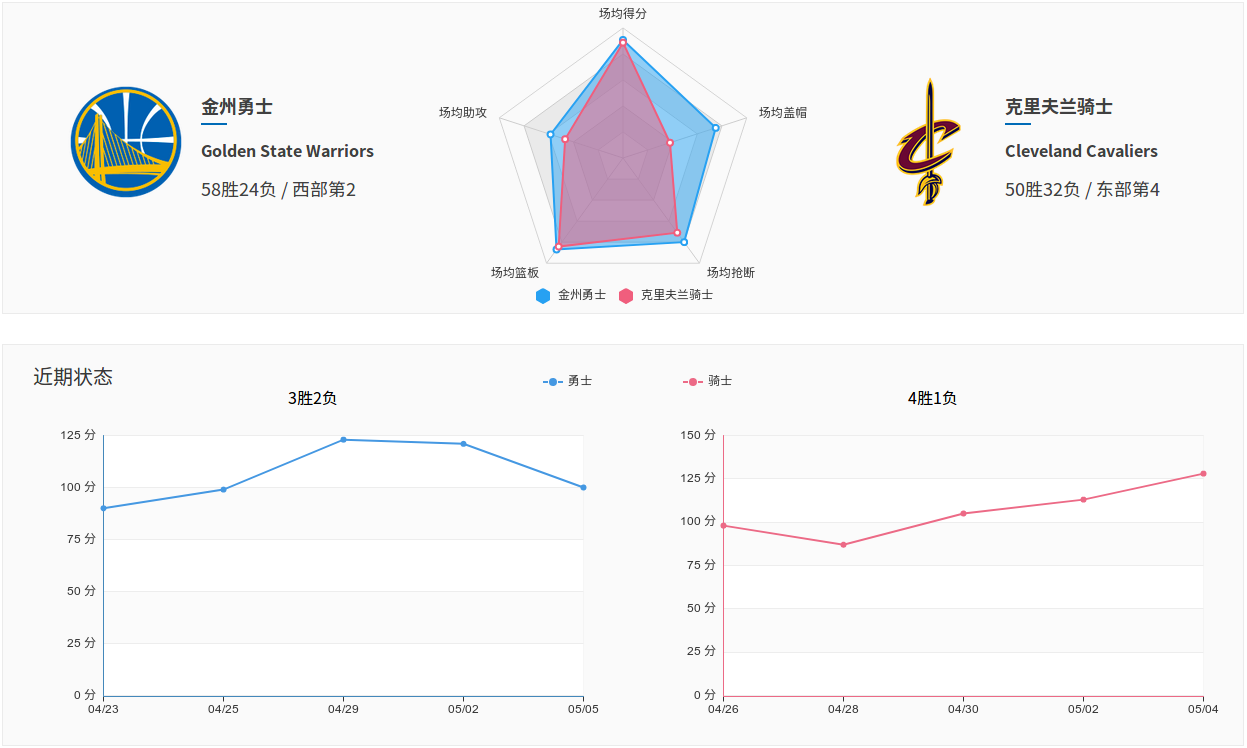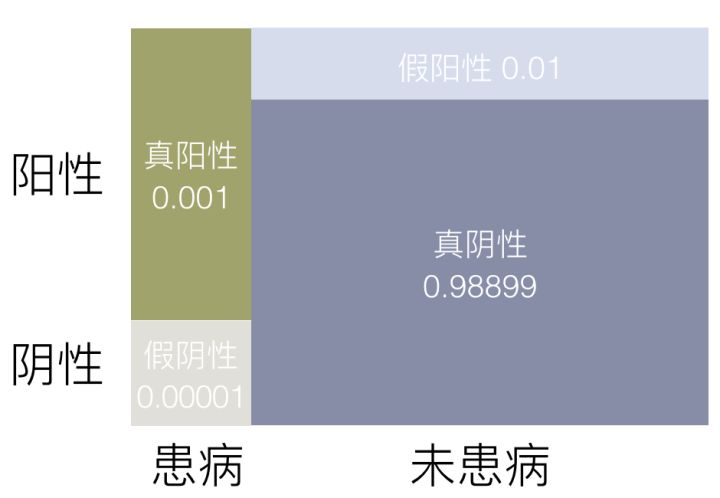# 趣味引入

我们在判断一件事情发生与否时，往往考虑到很多方面，不会单纯的瞎猜而是会通过已有的知识去预测这件事情发生的概率。比如最受广大男性同胞喜爱的足球比赛某只队伍的输赢问题上， 我们在判断这只队伍的输赢时。如果就论比赛胜负的话，要么输要么赢，输赢的概率各是$$\frac{1}{2}$$。但是你会说德国踢日本双方输赢一样吗？ 显然不是，我们分析一支队伍的输赢往往会考虑队伍的人员配置，球队是否是在主场比赛，球队的近期表现和队伍凝聚力等等因素。当我们知道越多的这些影响比赛的因素， 我们就可以越大胆的预测这场足球比赛到底谁会赢下。NBA季后赛一旦开打，ESPN就会在一堆数据基础上预测各轮比赛的胜负和总冠军球队的概率， 这些预测的前提都是来自于大量数据的指向。利用已有的知识去评估事物发生的概率就是贝叶斯式的思考方式。充分利用已有知识分析事物是非常重要的，这个也应用到了很多方面，我们看一个具体的例子。这个例子可能大家之前看过就是疾病检测的一个例子， 假设某种疾病在所有人群中的感染率是0.1%，医院现有的技术对于该疾病检测准确率为 99%（已知患病情况下， 99% 的可能性可以检查出阳性； 正常人 99% 的可能性检查为正常），如果从人群中随机抽一个人去检测，医院给出的检测结果为阳性，那么这个人实际得病的概率是多少？

会不会有人说当然是99%了，但是你要注意疾病监测99%是在确定病人患病的情况下有99%的可能性是阳性的。我们现在问的是当检测结果为阳性时，这个人患病的概率？

我们说了既然是利用贝叶斯方式去思考这类问题当然要充分利用我们已知的知识喽。我们从信息中知道，在茫茫人群中仅有0.1%的患病率，但是拉来这个做测试的人， 偏偏检测结果为阳性，然而在患病人群中有99%的人检测结果也为阳性。这就是我们知道的所有信息了，当然我们再剥离出相关联的一些信息，我们用 A 表示这个人患有该疾病， 用 B 表示医院检测的结果是阳性，$$\bar A$$表示A的补集也就是这个人未得该病，可以整理得到：

$$P(A)=0.001$$，被检测者患病的概率

$$P(\bar A)=0.999$$，被检测者未患病的概率

$$P(B\vert A)=0.99$$，已知患病的情况下检测为阳性的概率

$$P(B\vert \bar A)=0.01$$，已知未患病的情况下检测为阳性的概率

有了这些已有的知识，我们就需要一套理论去利用这些知识最终确定到底检测到阳性的人患病的概率是多少，这个还是很重要的，毕竟你要知道到底得病的概率是多大。

# 预备知识

在真正说贝叶斯公式前，我们需要对概率论中的几个知识点要很清楚，那就是一些概率的定义。

有这些知识，我们大胆的做如下尝试：

$P(A,B) = P(A|B) \cdot P(B) = P(B|A) \cdot P(A)$

通过移项，我们可以得到：

$P(A\vert B) = \frac{P(B|A) \cdot P(A)}{P(B)} (1)$

这个就是我们的贝叶斯公式的雏形，我们暂时不分析这个公式，我们来看看先验概率和后验概率。我们知道$$P(A\vert B)$$是在 B 发生的情况下 A 发生的可能性：

# 贝叶斯公式

有了上述的知识点，我们就可以来具体说说贝叶斯公式的具体了。我们上述大胆的尝试得到了公式1，这个公式1其实就是贝叶斯公式了，我们将它写的正规一点，普世一点。

$P(A_i \vert B) = \frac{P(B\vert A_i) \cdot P(A_i)}{\sum _{j=1}^n P(A_j) \cdot P(B\vert A_j)} (2)$

公式2更具有一般性，描述一个事件，往往会有相应的事件伴随着发生考虑的全面点，已有的知识充分利用上才是贝叶斯后验概率的精髓。

我们接下来为了分析的简单一些，都是用公式1作为基础分析展开。我们看公式1$$P(A\vert B) = \frac{P(B\vert A) \cdot P(A)}{P(B)}$$，我习惯这样来看贝叶斯公式， $$P(A\vert B) = P(A)\cdot \frac{P(B\vert A)}{P(B)}$$，这时候可以将贝叶斯公式看为两个部分，第一部分是先验概率P(A)，后一部分是“调整因子”。

我们详细点说就是把P(A)称为”先验概率”（Prior probability），即在B事件发生之前，我们对A事件概率的一个判断。$$P(A|B)$$称为”后验概率”（Posterior probability）， 即在B事件发生之后，我们对A事件概率的重新评估。$$\frac{P(B\vert A)}{P(B)}$$称为”可能性函数”（Likelyhood），这是一个调整因子，使得预估概率更接近真实概率。

所以，条件概率可以理解成下面的式子：

$后验概率 ＝ 先验概率 \times 调整因子 (3)$

这就是贝叶斯推断的含义。我们先预估一个”先验概率”，然后加入实验结果，看这个实验到底是增强还是削弱了”先验概率”，由此得到更接近事实的”后验概率”。

在这里，如果”可能性函数”$$\frac{P(B\vert A)}{P(B)}>1$$，意味着”先验概率”被增强，事件A的发生的可能性变大；如果”可能性函数”=1，意味着B事件无助于判断事件A的可能性； 如果”可能性函数”<1，意味着”先验概率”被削弱，事件A的可能性变小。

有了以上对贝叶斯公式的分析，我们回过头来看看之前提到的那个没有解决的检测阳性情况下得病概率的计算。回顾一下，我们用 A 表示这个人患有该疾病， 用 B 表示医院检测的结果是阳性，$$\bar A$$表示A的补集也就是这个人未得该病，道我们已经有了茫茫人海中得病概率P(A) = 0.001的先验概率,也有了得病情况下为阳性的概率 $$P(B\vert A)=0.99$$，在分析中我们知道被检测者未患病的概率$$P(\bar A)=0.999$$，已知未患病的情况下检测为阳性的概率$$P(B\vert \bar A)=0.01$$。 现在我们要计算的就是在阳性情况下得病的概率，即为在已知检测结果为阳性的情况下得病的后验概率$$P(A\vert B)$$是多少?

我们利用贝叶斯公式来算了：

$P(A|B) = P(A)\cdot \frac{P(B|A)}{P(B|A)\cdot P(A) + P(B|\bar A)\cdot P(\bar A)} = 0.001 \cdot \frac{0.99}{0.99 \times 0.001 + 0.01 \times 0.999} = 0.09016$

通过计算我们得到，在这个前提下即使你检测出的是阳性，你得这个病的概率也仅为9%左右，所以，在医学没达到完美的情况下都是存在着一定的误差的。 即使你查出了阳性你仍然有很大概率是没有得病的，为了确定往往需要复查，这种情况就是我们常常提及的假阳性，我们通过图看一下。从贝叶斯的角度来看，随意选取的一个被测者，由于信息并不充分，未检测之前有假阳性、真阳性、假阴性和真阴性四种可能，这些可能性由检测技术和该疾病的感染率决定， 当检测结果为阳性的时候，只剩下真阳性和假阳性两种可能，而真阳性的概率仅为假阳性的十分之一，贝叶斯公式在这里的实际意义是：

$P(A\vert B) = \frac{True Positive}{True Positive + False Positive}$

即使被医院检测为阳性，实际患病的概率其实还不到10%，有很大可能是假阳性，往往需要复检来确定是否真的患病，让我们再来计算初检和复检结果都为阳性时， 患病的可能性。假设两次检查的准确率相同，都是99%，这里令 B 为第一次检测结果为阳性，C 为第二次检测结果为阳性，A 为被检测者患病， 那么两次检测结果都是阳性患病的概率可以表示为：

$P(A\vert (B \cap C)) = P(A)\cdot \frac{P((B \cap C)|A)}{P((B \cap C)|A)\cdot P(A) + P((B \cap C)|\bar A)\cdot P(\bar A)}$

$$P(A)=0.001$$，被检测者患病的概率

$$P(\bar A)=0.999$$，被检测者未患病的概率

$$P((B \cap C)\vert A)=0.99 \times 0.99=0.9801$$，已知患病情况下连续两次检测结果为阳性的概率

$$P((B \cap C)\vert \bar A)=0.01 \times 0.01=0.0001$$，已知未患病情况下连续两次检测结果为阳性的概率

$P(A\vert (B \cap C)) \approx 0.9$